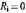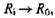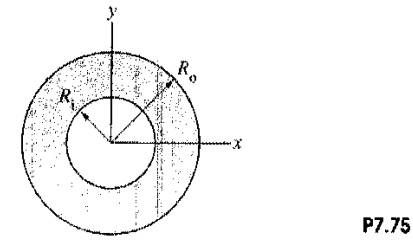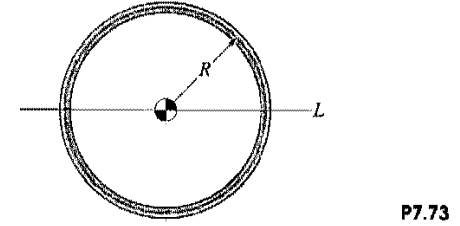### Create an Account

Home / Questions / The brass washer is of uniform thickness and mass m a Determine its mass moments of inert...

# The brass washer is of uniform thickness and mass m a Determine its mass moments of inertia about the x and z axes b Let and compare your results with the values given in Appendix C for a

The brass washer is of uniform thickness and mass m.

(a) Determine its mass moments of inertia about the x and z axes.

(b) Letand compare your results with the values given in Appendix C for a thin circular plate.

(c) Letand compare your results with the solutions of Problem 7.73.Problem 7.73.

A homogeneous, slender bar is bent into a circular ring of mass m and radius R. Determine the mass moment of inertia of the ring (a) about the axis through its center of mass that is perpendicular to the ring; (b) about the axis L.Jun 15 2020 View more View LessSubscribe To Get Solution